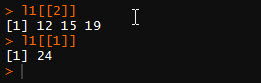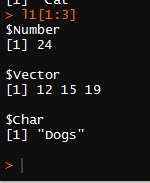# R: Working with lists

Lists in R allow you to store data of different types into a single data structure. While they are extremely useful, they can be a bit confusing to work with at first.

Let’s start by creating a list. The syntax is simple enough, just add list() around the elements you want to put in your list.

```l1 <- list(24, c(12,15,19), "Dogs")
l1```

Here is the output. Note we have 3 different groupings. 1 number, 1 vector (with 3 elements) and 1 characterYou can call on each element using the element names (found in the double brackets [[]])

```l1[]
l1[]```

Here are the resultsNow, what if you want to call 1 element from the vector in []

You do this by adding [] to the end of the line

`l1[]`

This will give you the 3rd number in the vector found in []To make it easier to work with lists, you can rename the elements.

```names(l1)  # shows NULL since the elements have no names
names(l1) = c("Number", "Vector", "Char")
names(l1) # now shows assigned names```

Now you can call on the list using the names.

```l1\$Char # will return "Dogs"

l1\$Vector #will return the second number in the vector in the list```

```rm(l1) #deletes list
l1 <- list(Number=24,Vector = c(12,15,19), Char="Dogs")```

You can add a new element to you list via number

`l1[] = "New Element"`

Even better, you can add via new name

`l1\$Char2 <- "Cat"`

Now let’s look at our listNow we can use the names() function to give element 4 a name, or we can just get rid of it.

To delete an elements, use NULL

`l1[] <- NULL`

Now the last thing we will cover is how to subset a list

`l1[1:3] # gives us elements 1 -3`We want to pick some elements out of order

```l1[c(2,4)]
l1[c("Number","Char")]```